### DiagonalA diagonal is a line joining two nonconsecutive vertices of a polygon or polyhedron. Informally, any sloping line is called diagonal. The word `diagonal` derives from the ancient Greek διαγώνιος diagonios, `from angle to angle` (from διά- dia-, `through`, `across` and γωνία gonia, `angle`, related to gony `knee`); it w...
Found on http://en.wikipedia.org/wiki/Diagonal

### Diagonal• (n.) A right line drawn from one angle to another not adjacent, of a figure of four or more sides, and dividing it into two parts. • (n.) A diagonal cloth; a kind of cloth having diagonal stripes, ridges, or welts made in the weaving. • (a.) Joining two not adjacent angles of a quadrilateral or multilateral figure; running across f...
Found on http://thinkexist.com/dictionary/meaning/diagonal/

### diagonal<geometry> Joining two not adjacent angles of a quadrilateral or multilateral figure; running across from corner to corner; crossing at an angle with one of the sides. Diagonal bond Same as Cross bedding, under Cross. ... Origin: L. Diagonalis, fr. Gr. From to angle; through + an angle; perh. Akin to E. Knee: cf. F. Diagonal. ... Source: Webs...
Found on http://www.encyclo.co.uk/local/20973

### Diagonal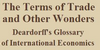1. In a matrix, the elements on a straight line from the top left to the bottom right, or occasionally from the bottom left to the top right. 2. In an Edgeworth box, the straight line from the bottom left corner to the top right. Along the diagonal, the ratios of allocations of the two agents (industries or consumers) are constant and equal. 3. In....
Found on http://www-personal.umich.edu/~alandear/glossary/d.html

### Diagonal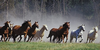3. In dressage tests, a line crossing the center of the competition ring running from one end corner to the opposite end corner. The diagonal is also used in some driving competition as the route for competitors to safely change direction in a ring or arena when there are a large number of entries.
Found on http://en.wikipedia.org/wiki/Glossary_of_equestrian_terms

### diagonalnoun an oblique line of squares of the same color on a checkerboard; `the bishop moves on the diagonals`
Found on https://www.encyclo.co.uk/local/20974

### DiagonalDi·ag'o·nal adjective [ Latin diagonalis , from Greek ... from to angle; dia` through + ... an angle; perhaps akin to English knee : confer French diagonal .] (Geom.) Joining two not adjacent angles of a quadrilateral or multilateral figure; running across from ...
Found on http://www.encyclo.co.uk/webster/D/56

### Diagonal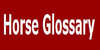A pair of legs at the trot, such as the right front and the left hind When posting, the rider sits as the inside hind hits the ground or "rise and fall with the (front) leg on the wall" Riding across the diagonal is a maneuver from one corner of an arena to another through the center
Found on http://www.gaitedhorses.net/Articles/HorseGlossary.html

### diagonal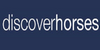a pair of legs moving in unison at the trot (e.g. right front, left hind). A correctly posting rider (said to be
Found on http://www.discoverhorses.com/resources/glossary-of-horse-terminology-horse

### diagonal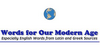diagonal 1. Extending between nonadjacent angles in a polygon figure. 2. Having slanting marks, lines, etc.
Found on http://www.wordinfo.info/words/index/info/view_unit/930/

### Diagonal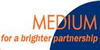For 4:3 formats (SXGA, XGA, SVGA) the image width is= diagonal : 1,25 see image size
Found on https://www.encyclo.co.uk/local/20955

### Diagonal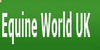Reference to the horse moving its leg in diagonal pairs whilst trotting.
Found on http://www.equine-world.co.uk/riding_horses/glossary.htm

### Diagonal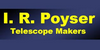See 'Star diagonal'
Found on http://www.irpoyser.co.uk/glossary.php

### diagonal[adj] - at an angle 2. [n] - (geometry) a straight line connecting any two vertices of a polygon that are not adjacent 3. [n] - a line or cut across a fabric that is not at right angles to a side 4. [n] - an oblique line of squares of the same color on a checkerboard 5. [n] - (mathematics) a set of entries in a square matrix ...
Found on http://www.webdictionary.co.uk/definition.php?query=diagonal

### diagonal• A line that joins any two vertices of a polygon, if the vertices are not next to each other; or aline that joins two vertices of a polyhedron that are not on the same face. • GEOMETRY • The small flat mirror used near the upper end of a Newtonian telescope to ...
Found on http://www.daviddarling.info/encyclopedia/D/diagonal.html
No exact match found